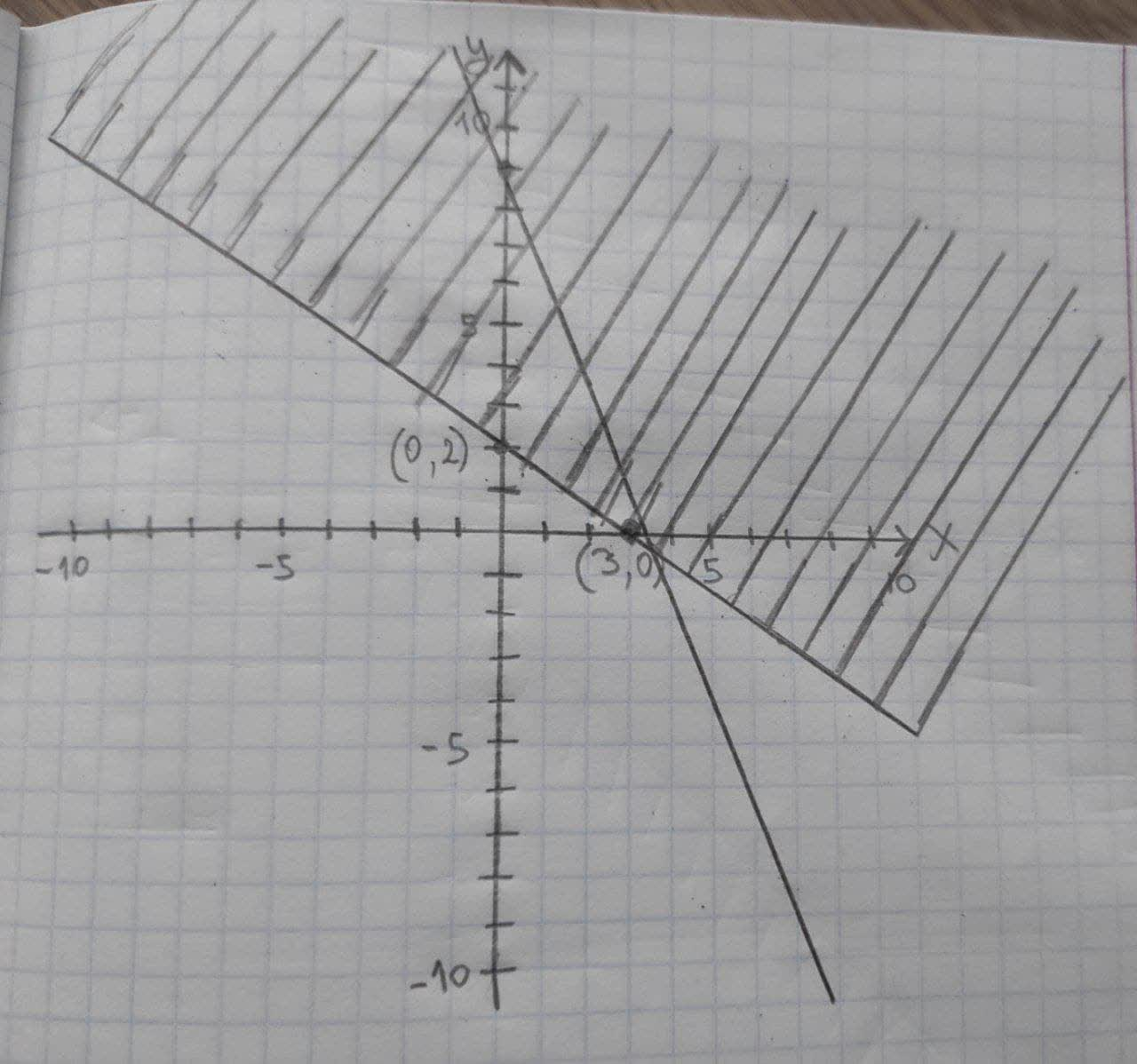# Graph the solution set of the inequality or system of inequalities. {(x>=0 and y>=0),(3x+y<=9),(2x+3y>=6):}Jason Farmer 2021-03-05 Answered
Graph the solution set of the inequality or system of inequalities.
$\left\{\begin{array}{c}x\ge 0\phantom{\rule{1em}{0ex}}\text{and}\phantom{\rule{1em}{0ex}}y\ge 0\\ 3x+y\le 9\\ 2x+3y\ge 6\end{array}$
You can still ask an expert for help

## Want to know more about Inequalities systems and graphs?

• Questions are typically answered in as fast as 30 minutes

Solve your problem for the price of one coffee

• Math expert for every subject
• Pay only if we can solve itJaylen Fountain
The graph of the inequality: